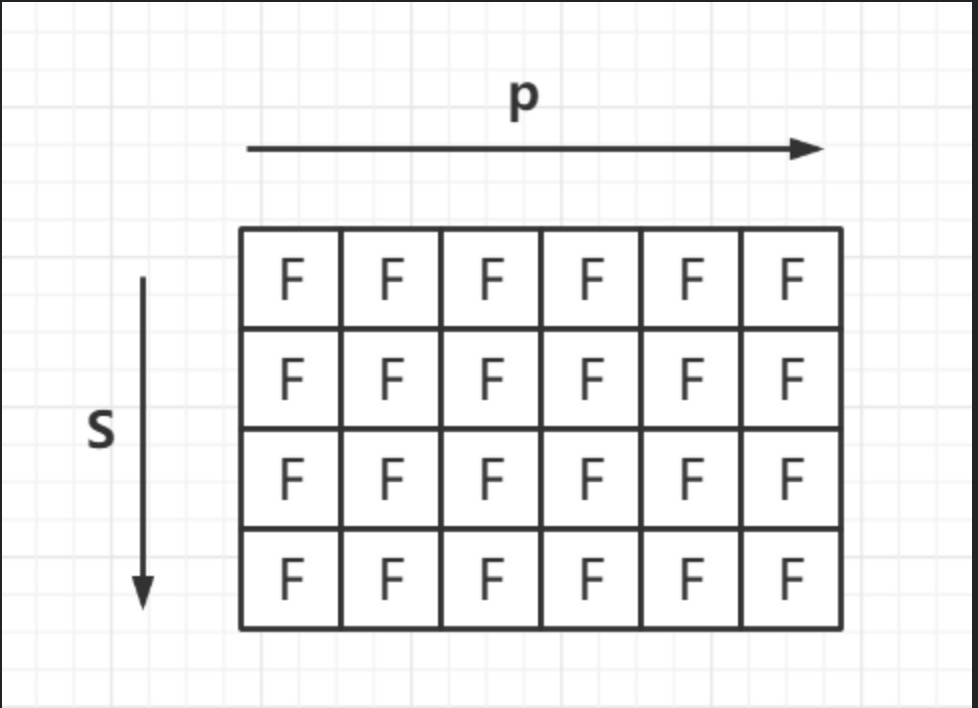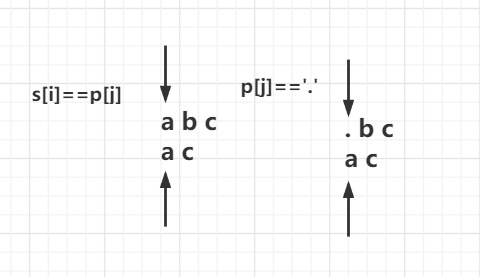# 10-正则表达式

## 定义状态

• 定义动态数组$dp[m][n]$ （n为字符串p的长度+1，m为字符串s的长度+1）
• 为什么要加1
• 因为我们还要处理空字符串的情况，比如p为空，s为空，或者p为空，s不为空
• $dp[m][n]$ 的含义：p的前$n-1$ 个字符能否匹配s的前$m-1$个字符
• 为什么是n-1和m-1?
• 因为动态数组里面加了一列和一行空字符的匹配情况，故需要-1才能对应相应字符串## 确定动态转移方程

• 当 $s[i]=p[j] \ || \ p[j]==’.’$ （即正好能够匹配或者相对应的是一个 $.$）• 当 $p[j] ==’‘$ （即匹配到了万能字符 ）

两种情况分别对应的处理方式为：

如果 $*$ 的前一个字符正好对应了$s$，状态转移过程为：$dp[r][c] = dp[r-1][c]$

如果是$*$的前一个字符为 $.$ 那么只需看$.$的前面字符匹配情况，状态转移过程为: $dp[r][c]=dp[r][c-2]$

• 首先我们要确定 $dp$，当p为空，s为空时，肯定匹配成功。那么$dp=true$

• 当 p 为空字符串，而s不为空时，dp数组必定为False，正好初始化dp数组的时候设置的是False；即dp数组的第一列为False可以确定

• 当s为空字符串，而p不为空时，我们无需判断p里面的第一个值是否为””，如果为””,那肯定匹配不到为Fasle,原数组正好是Fasle，所以直接从2开始判断即可。如果遇到了*,只要判断其对应的前面两个元素的dp值